StatLect

# Almost sure

In probability theory, a property is said to hold almost surely if it holds for all sample points, except possibly for some sample points forming a subset of a zero-probability event.

If an event happens almost surely, then it is called an almost sure event.## Synonyms and acronyms

The abbreviations a.s. (almost sure or almost surely) and w.p. 1 (with probability 1) are used very frequently.

## Definition

The concept is defined as follows.

Definition Letbe a sample space. Letbe the set of its points satisfying a given property: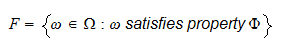Propertyis said to be an almost sure property if the setof all points that do not satisfy propertyis included in a zero-probability event, i.e.,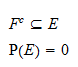Remember that not all subsets of the sample space are necessarily considered events (see the lecture entitled Probability). Therefore, the setneeds not necessarily be an event. In case it is, it is called an almost sure event.

## Example

Suppose the sample space is the unit interval, that is,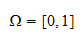and suppose that the set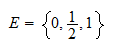is a zero-probability event: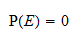Now consider the function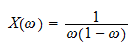The function is well-defined on the interior ofbut not at its endpoints (and), where it tends to infinity. Can we say thatis almost surely well-defined on? Define the set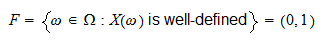Then the complement ofis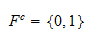Clearly, we have that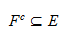and we know thatis a zero-probability event. As a consequence, we can say thatis almost surely well-defined on.

## More details

A more articulated explanation, as well as an extensive discussion of the subtleties of the concept of zero-probability event, is provided in the lecture entitled Zero-probability events.